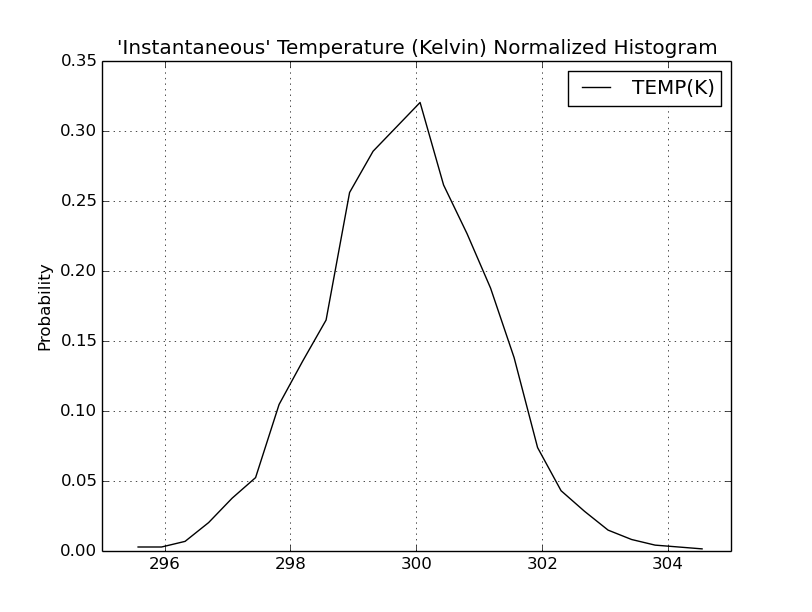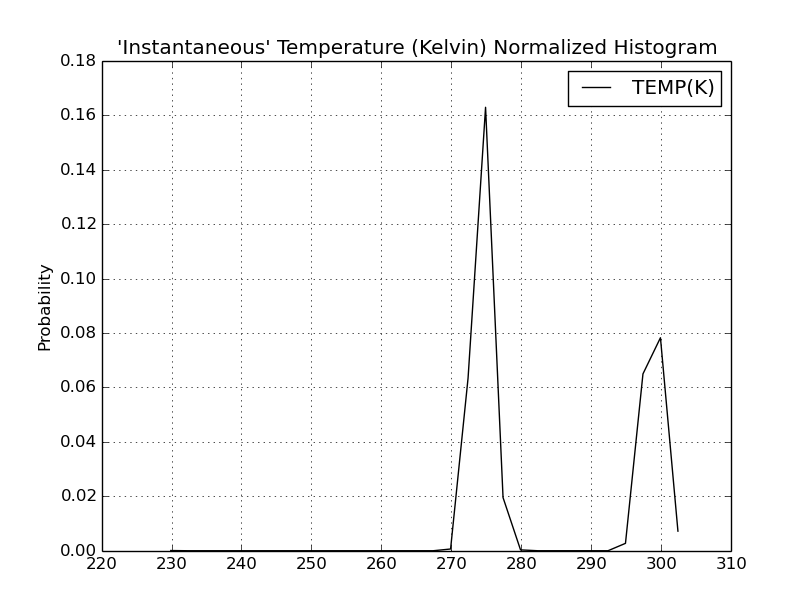# Re: [AMBER] constant pH md

From: Maciej Maciejczyk <maciej.maciejczyk.uwm.edu.pl>
Date: Thu, 11 Jan 2018 13:52:16 +0100

Dear Vinicius,

Thank you for the reply, but I do not think it is a problem with
equilibration. The problem must be related to constant pH simulations.
Please find attached two histograms of temperature - one from the
equilibration phase and another from cpH simulation run. Probably it is
better visualization of the problem than fragment of output file which I
sent previously.

Best regards,

Maciej

On 11.01.2018 13:04, Cruzeiro,Vinicius Wilian D wrote:
> Dear Maciej,
>
> The temperature is expected to fluctuate throughout the simulation, regardless of solvent relaxation. When the simulation finishes, you will be able to check the average temperature at the bottom of the output file. See if it is close to 300 K. If not, check the behavior during your thermalization/equilibration stages. Here are some things to consider: make sure you are running long enough and that your water buffer is big enough. You possibly did a NPT equilibration, so check if your density is close to 1.0 there. Also, double check the values of restraint_wt and restraintmask you are using on each stage.
>
> I hope this helps,
> Best,
>
>
> Vinícius Wilian D Cruzeiro
>
> PhD Candidate
> Department of Chemistry, Physical Chemistry Division
> University of Florida, United States
>
> Voice: +1(352)846-1633<tel:+1(352)846-1633>
>
> On Jan 11, 2018, at 2:45 AM, Maciej Maciejczyk <maciej.maciejczyk.uwm.edu.pl<mailto:maciej.maciejczyk.uwm.edu.pl>> wrote:
>
> Dear amber users,
>
> I have a question regarding explicit solvent constant pH simulation of
> protein. The problem is that mean temperature printed in output file is
> well below requested temperature of 300 K. I assume that it has
> something to do with solvent relaxation step during which the protein is
> frozen.
>
> The question is whether it is only a problem with temperature showed in
> the output file, or is it a deeper problem of incorrect coupling with
> the thermal bath. Can I just ignore this issue?
>
> Here is fragment of output:
>
> NSTEP = 11000 TIME(PS) = 4422.000 TEMP(K) = 275.67 PRESS
> = 0.0
> NSTEP = 12000 TIME(PS) = 4424.000 TEMP(K) = 276.72 PRESS
> = 0.0
> NSTEP = 13000 TIME(PS) = 4426.000 TEMP(K) = 272.92 PRESS
> = 0.0
> NSTEP = 14000 TIME(PS) = 4428.000 TEMP(K) = 299.79 PRESS
> = 0.0
> NSTEP = 15000 TIME(PS) = 4430.000 TEMP(K) = 274.19 PRESS
> = 0.0
> NSTEP = 16000 TIME(PS) = 4432.000 TEMP(K) = 301.80 PRESS
> = 0.0
> NSTEP = 17000 TIME(PS) = 4434.000 TEMP(K) = 274.60 PRESS
> = 0.0
> NSTEP = 18000 TIME(PS) = 4436.000 TEMP(K) = 276.55 PRESS
> = 0.0
> NSTEP = 19000 TIME(PS) = 4438.000 TEMP(K) = 298.17 PRESS
> = 0.0
> NSTEP = 20000 TIME(PS) = 4440.000 TEMP(K) = 275.76 PRESS
> = 0.0
> NSTEP = 21000 TIME(PS) = 4442.000 TEMP(K) = 274.77 PRESS
> = 0.0
> NSTEP = 22000 TIME(PS) = 4444.000 TEMP(K) = 300.32 PRESS
> = 0.0
> NSTEP = 23000 TIME(PS) = 4446.000 TEMP(K) = 276.98 PRESS
> = 0.0
> NSTEP = 24000 TIME(PS) = 4448.000 TEMP(K) = 298.93 PRESS
> = 0.0
> NSTEP = 25000 TIME(PS) = 4450.000 TEMP(K) = 274.52 PRESS
> = 0.0
> NSTEP = 26000 TIME(PS) = 4452.000 TEMP(K) = 274.62 PRESS
> = 0.0
> NSTEP = 27000 TIME(PS) = 4454.000 TEMP(K) = 274.36 PRESS
> = 0.0
> NSTEP = 28000 TIME(PS) = 4456.000 TEMP(K) = 299.36 PRESS
> = 0.0
> NSTEP = 29000 TIME(PS) = 4458.000 TEMP(K) = 274.12 PRESS
> = 0.0
> NSTEP = 30000 TIME(PS) = 4460.000 TEMP(K) = 277.08 PRESS
> = 0.0
> NSTEP = 31000 TIME(PS) = 4462.000 TEMP(K) = 276.03 PRESS
> = 0.0
> NSTEP = 32000 TIME(PS) = 4464.000 TEMP(K) = 276.08 PRESS
> = 0.0
> NSTEP = 33000 TIME(PS) = 4466.000 TEMP(K) = 276.44 PRESS
> = 0.0
> NSTEP = 34000 TIME(PS) = 4468.000 TEMP(K) = 299.66 PRESS
> = 0.0
>
> ... and here is the input:
>
> Explicit solvent constant pH MD
> &cntrl
> imin=0, irest=1, ntx=5, ntxo=2,
> ntpr=1000, ntwx=1000, nstlim=5000000,
> dt=0.002, ntt=3, tempi=300,
> temp0=300, gamma_ln=5.0, ig=-1,
> ntc=2, ntf=2, cut=10, iwrap=1,
> ioutfm=1, icnstph=2, ntcnstph=100,
> solvph=6.6, ntrelax=100, saltcon=0.1,
> /
>
>
> --
> *******************************************
> Maciej S. Maciejczyk, PhD
> Division of Physics and Biophysics
> Faculty of Food Sciences
> University of Warmia and Mazury in Olsztyn
> ul. Oczapowskiego 4
> 10-719 Olsztyn
> Poland
> *******************************************
>
>
> _______________________________________________
> AMBER mailing list
> AMBER.ambermd.org<mailto:AMBER.ambermd.org>
> https://urldefense.proofpoint.com/v2/url?u=http-3A__lists.ambermd.org_mailman_listinfo_amber&d=DwIGaQ&c=pZJPUDQ3SB9JplYbifm4nt2lEVG5pWx2KikqINpWlZM&r=LIQu8OlVNKmzfbMg9_5FnKrt9-DrdQBJXyFyocKAWXc&m=789oTAq_BOpAffU_qdvMdllg-hSzmP2DaaLeRS1F8dY&s=pzBH5vT-SLv6So_Icz2hRbS-QWhp-pfuL25VmRiis5c&e=
> _______________________________________________
> AMBER mailing list
> AMBER.ambermd.org
> http://lists.ambermd.org/mailman/listinfo/amber

```--
*******************************************
Maciej S. Maciejczyk, PhD
Department of Physics and Biophysics
Faculty of Food Sciences
University of Warmia and Mazury in Olsztyn
ul. Oczapowskiego 4
10-719 Olsztyn
Poland
*******************************************

```

_______________________________________________
AMBER mailing list
AMBER.ambermd.org
http://lists.ambermd.org/mailman/listinfo/amber(image/png attachment: eq.png)(image/png attachment: mdrun.png)

Received on Thu Jan 11 2018 - 05:00:05 PST
Custom Search